# The Preparation of Fourier Series Matlab

In some cases, this Fourier series Matlab sample can help students to deal with their own homework. From the point of view of a student, the sample is a great helper if you have similar homework. However, if you don’t want to do your assignment on your own, you can leave it to AssignmentShark.com. We have already helped numerous students with different kinds of assignments. You can check out our blog to see more samples in order to understand what type of tasks our experts can solve.

In the course of completing your order, you have the opportunity to contact the expert directly via chat. You can plan your time as you want while we are completing your order. Your problem solution will be carried out by a professional in your sphere of study. The assignment will be created according to your requirements and academic standards. Due to the fact that homework can be difficult for an average student, we have decided to offer our help to those in need. The assignment help online will be executed in the fastest possible time, so you will receive a completed order even before the deadline.

Using Fourier series methods, solve the heat equation following the inhomogeneous initial boundary value problem using Matlab: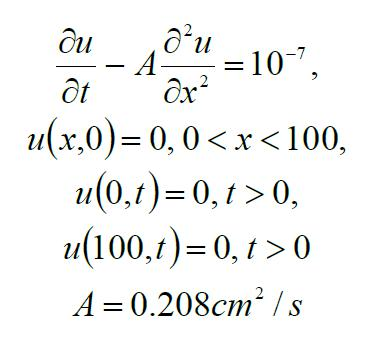Solution:

First we need to set the constant: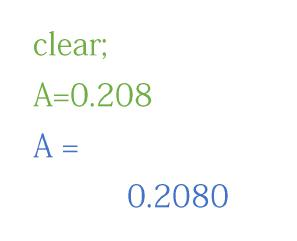The solution to this problem can be written as follows: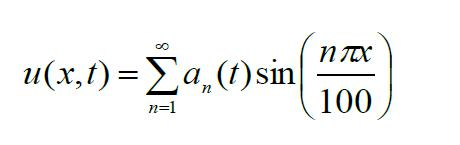Where the coefficient an (t) satisfies the initial value problem: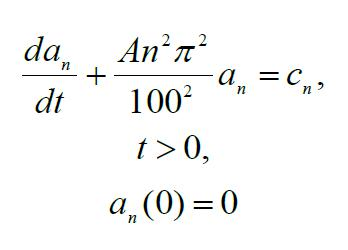The values c1, c2, c3 … are the Fourier sine coefficients of the constant function 10-7: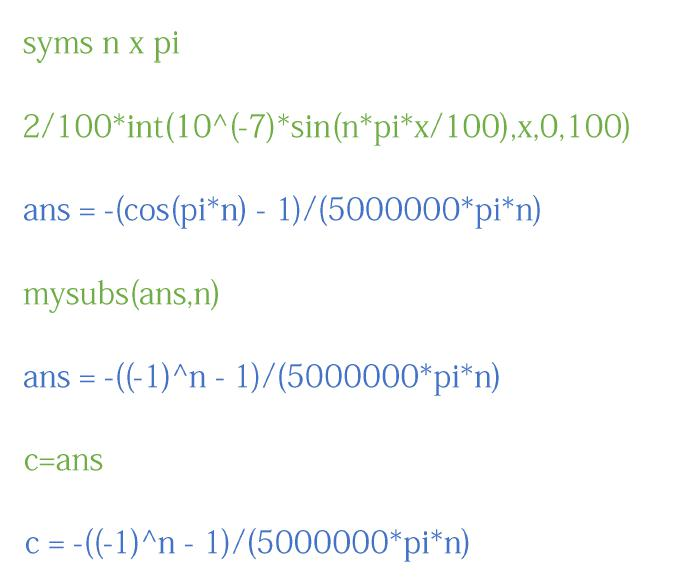The coefficient an (t) is given by the following formula: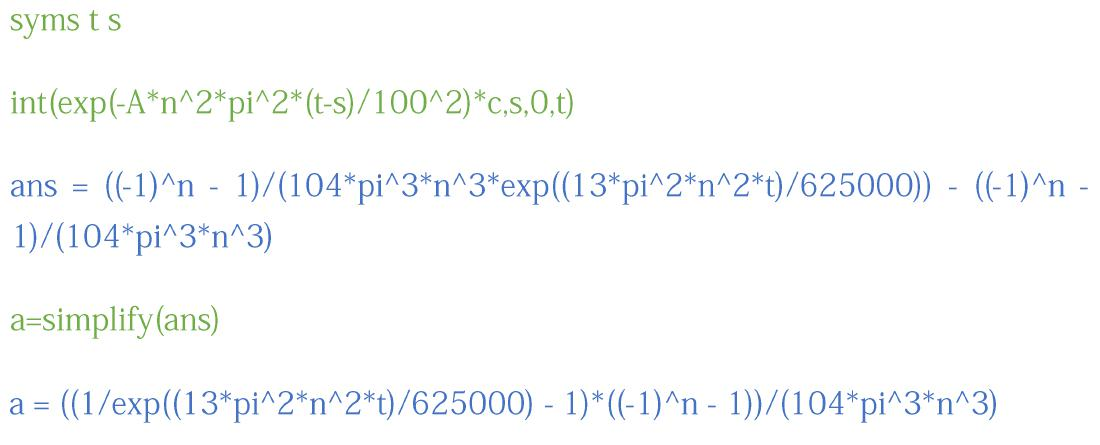Next we can define the partial Fourier series of the solution:

S=symsum(a*sin(n*pi*x/100),n,1,10);

Fourier series is required for a qualitatively correct solution.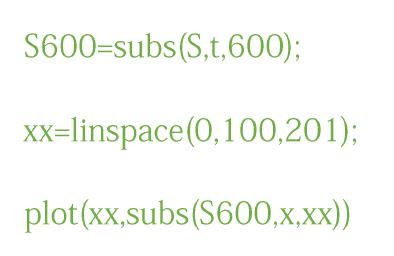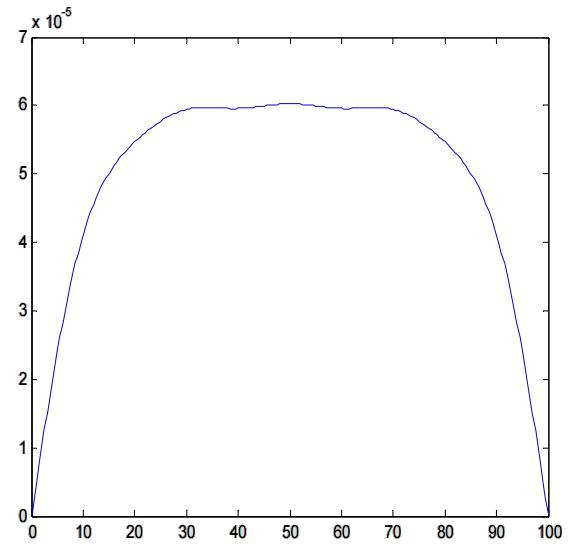The wiggles in the graph suggest that 10 terms is not enough for a correct graph.

Therefore, we will try again with 20 terms: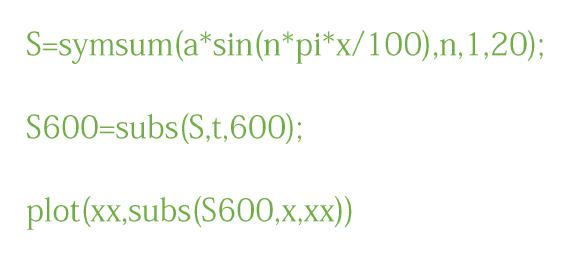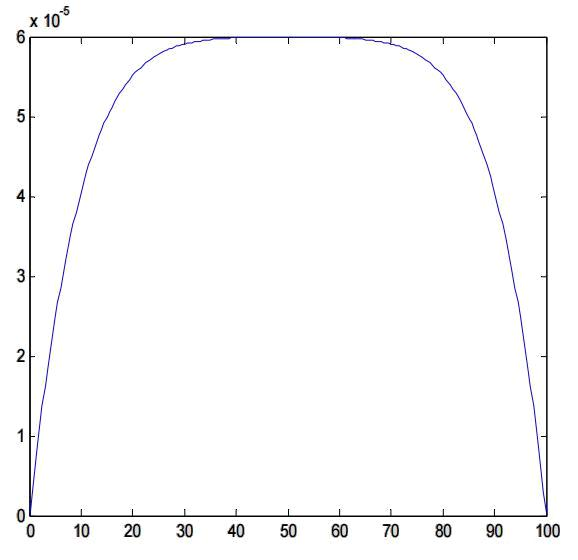Now let’s try with 40 terms: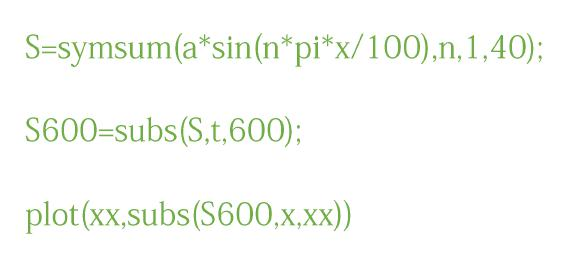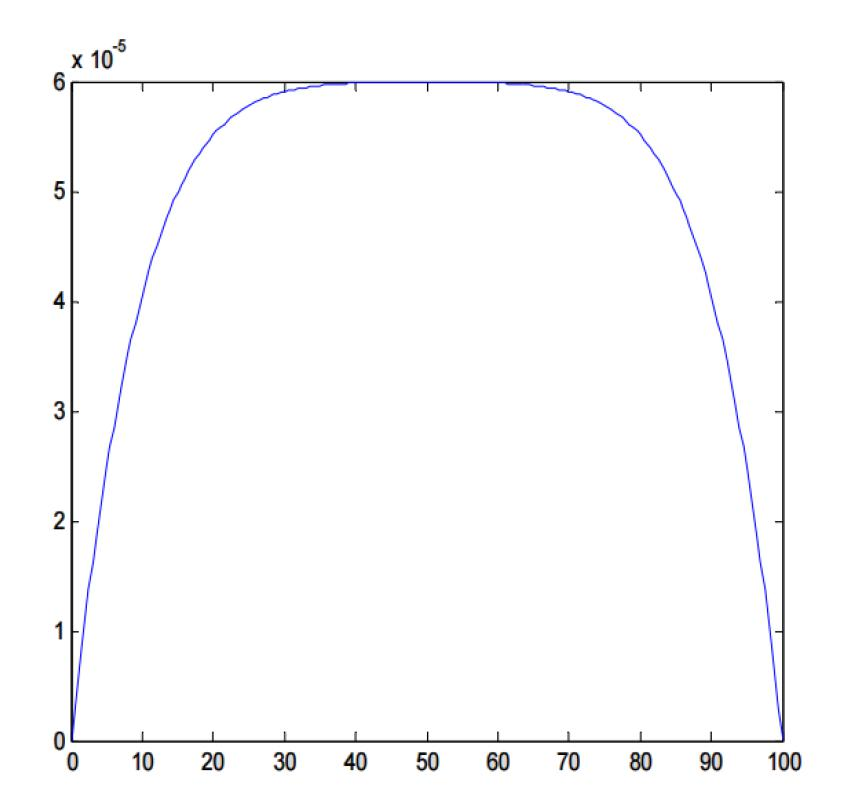It appears that 20 terms is enough for a qualitatively correct graph at t=600.